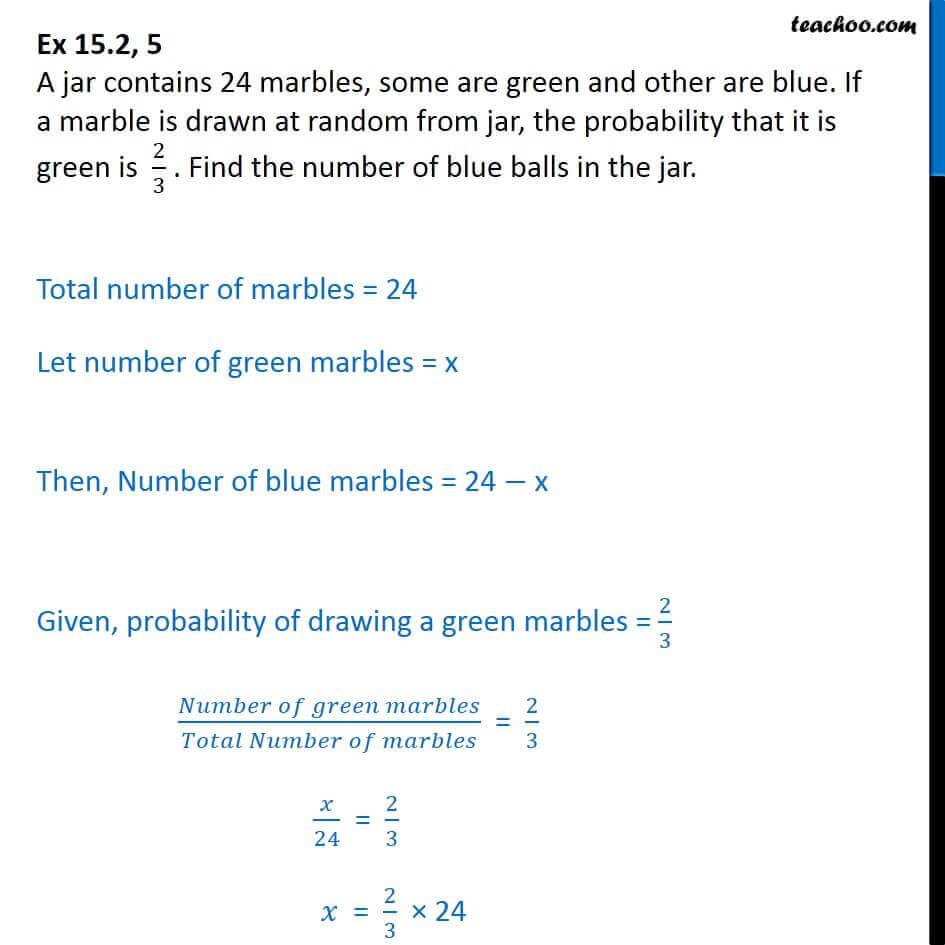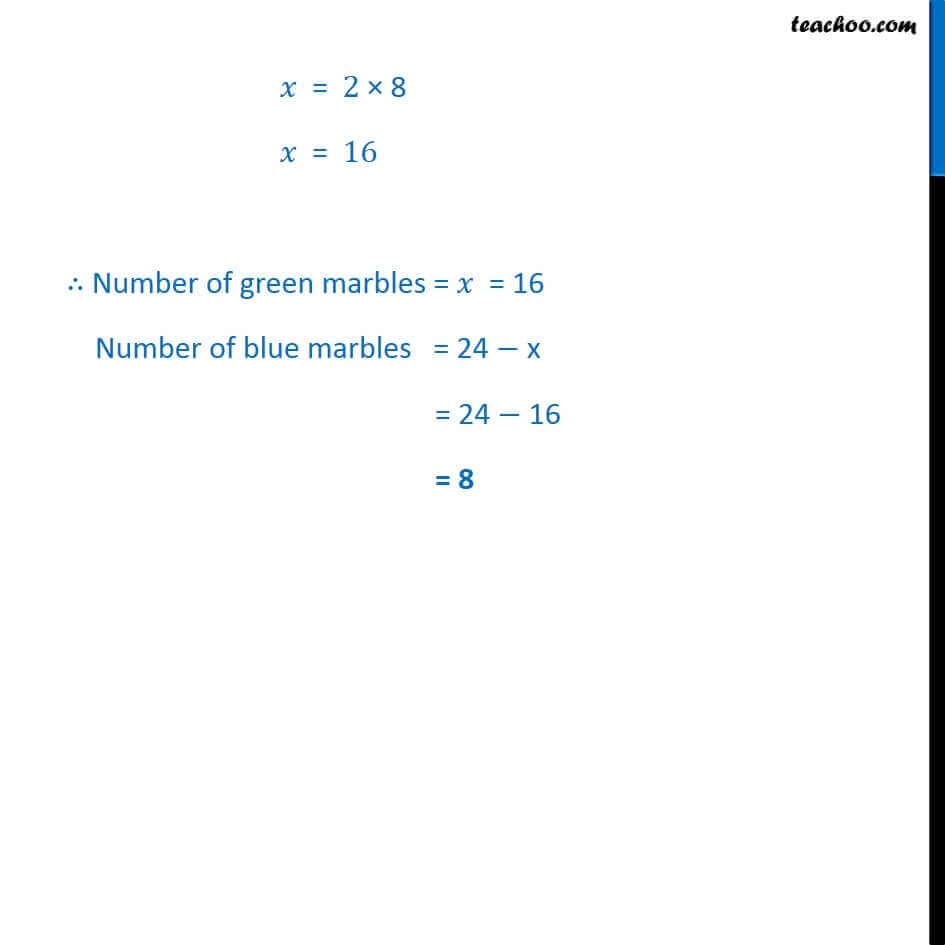Important Probability Questions

Chapter 14 Class 10 Probability
Serial order wiseLearn in your speed, with individual attention - Teachoo Maths 1-on-1 Class

### Transcript

Question 5 A jar contains 24 marbles, some are green and other are blue. If a marble is drawn at random from jar, the probability that it is green is 2/3 . Find the number of blue balls in the jar. Total number of marbles = 24 Let number of green marbles = x Then, Number of blue marbles = 24 x Given, probability of drawing a green marbles = 2/3 ( )/( ) = 2/3 /24 = 2/3 = 2/3 24 = 2 8 = 16 Number of green marbles = = 16 Number of blue marbles = 24 x = 24 16 = 8